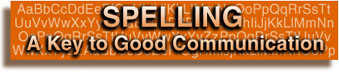### Lesson Two - "s" ending

The letter s is added to words to make them plural. It is also added to verbs to show present time. Below are three basic rules that outline how to add the s ending to words. There are also several special verbs that don't follow these rules. Study each rule and then complete the practice exercise.

 Rule 1: add the letter s to most words want + s = wants     like + s = likes top + s = tops Rule 2: add es after ch, sh, s, z or x lunch + es = lunches     wax + es = waxes Rule 3: if a word ends in y after a consonant, change y to i and add es ferry + s = ferries     carry + s = carries caution: this rule only applies if the y follows a consonant if the y follows a vowel (a e i o u), follow Rule 1 and just add s pays, plays, strays Special verbs : go + s = goes    do + s = does    have + s = has